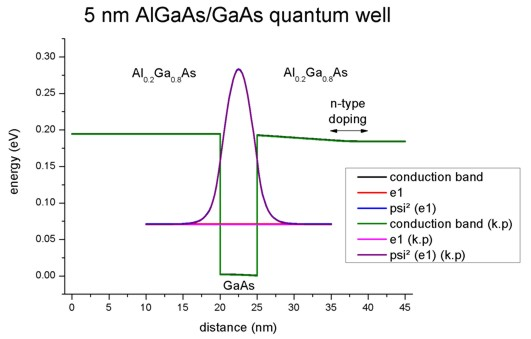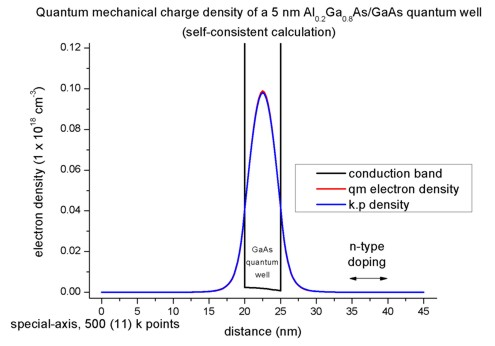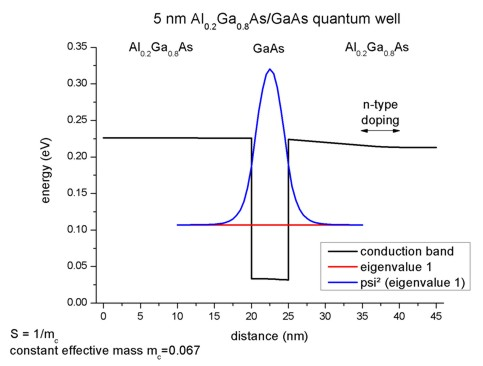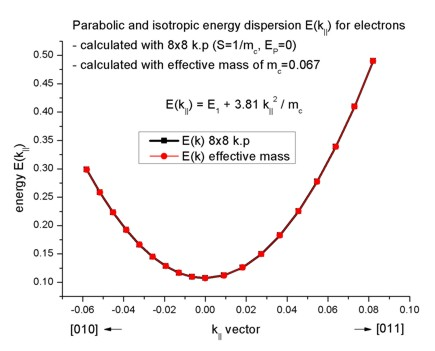nextnano.com  GUI: nextnanomat  Tool: nextnano++  Tool: nextnano³  Tool: nextnano.NEGF  Download | Search | Copyright | News | Publications  * password protected nextnano³ software1D single-band vs. k.p

# nextnano3 - Tutorial

## Single-band ('effective-mass') is a special case of 8-band k.p ('8x8kp') if the electrons are decoupled from the holes

Author: Stefan Birner

If you want to obtain the input file that is used within this tutorial, please contact stefan.birner@nextnano.de.
```-> effective_mass_vs_8x8kp_sg_1D.in -> effective_mass_vs_8x8kp_kp_1D.in -> effective_mass_vs_8x8kp_density_sg_1D.in -> effective_mass_vs_8x8kp_density_kp_1D_gendos.in -> effective_mass_vs_8x8kp_density_kp_1D_simple.in -> effective_mass_vs_8x8kp_density_kp_1D_special.in -> effective_mass_vs_8x8kp_same_mass_sg_1D.in -> effective_mass_vs_8x8kp_same_mass_kp_1D_gendos.in -> effective_mass_vs_8x8kp_same_mass_kp_1D_simple.in -> effective_mass_vs_8x8kp_same_mass_kp_1D_special.in```

This tutorial is a bit complicated and contains probably too much details. Nevertheless, an experienced user might want to understand in detail how certain properties are calculated in order to convince himself about the correctness of the nextnano³ results.

## 1) Single-band ('effective-mass') is a special case of 8-band k.p ('8x8kp') if the electrons are decoupled from the holes

``` -> 1Deffective_mass_vs_8x8kp_sg.in -> 1Deffective_mass_vs_8x8kp_kp.in```

The 8-band k.p parameters are specified as follows:

``` 8x8kp-parameters = L'   M    N'       ! L',M,N' [hbar^2/2m] (--> divide by hbar^2/2m) old version:        B    P    S        ! B       [hbar^2/2m], P   [eV * Angstrom], S []                     B    E_P  S        ! B       [hbar^2/2m], E_P [eV]           , S []```

To decouple the electrons from the holes one has to perform the following modifications on the 8-band k.p material parameters``` S ```and` P`:

• `S` = 1 / ``` me```   : ``` me``` = electron mass at the the Gamma point
```conduction-band-masses =  me    me   me                           ...  ...  ...                           ...  ...  ...```
• `E_P` = 0          : (equivalent to P = 0; Ep = Kane momentum matrix element; Ep = 2m0/hbar² * P²)
• The hole parameters `L'`, ``` M,``` `N'` can be arbitrary. However, they should be chosen so that spurious solutions do not arise.

With these modifications, both eigenvalues and wave functions must be identical for the cases
``` \$quantum-model-electrons   ...   model-name                     = effective-mass  ! ```single-band Schrödinger equation```   model-name                     = 8x8kp           ! ```multi-band (8x8) k.p Schrödinger equation

Note that for` effective-mass `the eigenvalues are two-fold degenerate due to spin. So we take twice as much in the case of k.p:
```  number-of-eigenvalues-per-band = 4               ! ```single-band Schrödinger equation```   number-of-eigenvalues-per-band = 8               ! ```multi-band (8x8) k.p Schrödinger equation

As an example we will take a simple unstrained AlAs/GaAs/AlAs quantum well structure where AlAs acts as the barrier material.
The conduction band offset is calculated as follows: 4.049 - 2.979 = 1.07 eV
```  conduction-band-energies = 4.049d0   ...      ...     ! ``` (AlAs)```   conduction-band-energies = 2.979d0   ...      ...     ! ```(GaAs)

The conduction band effective masses at the Gamma point are:
```  conduction-band-masses   =  0.15d0   0.15d0   0.15d0  ! ```(AlAs)```                               ...      ...      ...                               ...      ...      ...   conduction-band-masses   =  0.067d0  0.067d0  0.067d0 ! ```(GaAs)```                               ...      ...      ...                               ...      ...      ...```

The relevant` S` = 1 / ``` me ```parameters are:
```  8x8kp-parameters         =  ...      ...      ...                               ...      ...      6.66666667d0  ! ```(AlAs)```   8x8kp-parameters         =  ...      ...      ...                               ...      ...      14.92537313d0 ! ```(GaAs)```  ```

### Results

• The following figure shows the conduction band profile and the three lowest (confined) eigenstates for k=0 including their charge densities (wave function Psi²) for a 5 nm AlAs/GaAs quantum well.The calculated eigenvalues can be found in the file``` Schroedinger_1band/ev1D_cb001_qc001_sg001_deg001_dir_Kx001_Ky001_Kz001.dat```:
```  e1 = 3.09100 eV   e2 = 3.43473 eV   e3 = 3.97526 eV ```Both,` effective-mass `and``` 8x8kp ```lead to exactly the same eigenvalues. Also the squared wave functions (Psi²) are identical. The squared wave functions are normalized in units of [1/nm] so that they integrate to 1.0 if the integration interval is between 10 nm and 35 nm. (The quantum cluster extends from 10 nm to 35 nm.) Note that the probabilities Psi² are shifted by the corresponding eigenvalues so that they fit nicely into the graph. To integrate Psi² one has to substract the appropriate eigenvalues in the data files.

New discretization routines:

```! 3) => effective-mass, box-integration, LAPACK-ZHBGVX   schroedinger-1band-ev-solv      = LAPACK-ZHBGVX   schroedinger-masses-anisotropic = box ! 4) => 8-band k.p, box-integration, LAPACK-ZHBGVX   schroedinger-kp-ev-solv         = LAPACK-ZHBGVX   schroedinger-kp-discretization  = box-integration   kp-cv-term-symmetrization       = yes   kp-vv-term-symmetrization       = yes ``` ```  e1 = 3.09100 eV   e2 = 3.43473 eV   e3 = 3.97526 eV `````` ! Note: Instead of 'LAPACK-ZHBGVX', one can also use 'arpack' or 'chearn' to get identical results. !       For '8x8kp' also 'LAPACK' works. !       'chearn' only works for 'effective-mass'. !       'arpack': "Error calculate_kp_eigenvalues: Eigenfunction zero." (eigenvalues are okay)```

## 2) Calculating the quantum mechanical density within the single-band approximation and 8-band k.p self-consistently

``` -> 1Deffective_mass_vs_8x8kp_density_sg.in    1Deffective_mass_vs_8x8kp_density_kp_gendos.in    1Deffective_mass_vs_8x8kp_density_kp_simple.in    1Deffective_mass_vs_8x8kp_density_kp_special.in```

Our input file contains a similar 5 nm quantum well but instead of AlAs we take Al0.2Ga0.8As as the barrier material. However, this time we include some n-type doping with a concentration of 1*1017 cm-3. Only the AlGaAs region between 35 and 40 nm is doped. The donor atom has a donor level of 0.007 eV below the conduction band.

We use` flow-scheme = 2`, i.e.
- we solve the Poisson equation including doping to obtain the electrostatic potential
- we use this electrostatic potential to solve Schrödinger's equation effective-mass (single-band) approximation
- we calculate the electron density (including quantum mechanical density) that enters the Poisson equation
- we solve the Poisson and Schrödinger equation self-consistently

The following figure shows the conduction band profile and the lowest eigestate as well as its probability amplitude. The chemical potential (i.e. the Fermi level) is not shown in the figure. It is equal to 0 eV. There are no further eigenstates that are confined inside the well. (The figure also shows the k.p results which are identical.)Note: In our example we take into account only the first quantized eigenstate. Usually one has to sum over all (occupied) eigenstates to calculate the quantum mechanical density.

The three-dimensional electron density of a 1D quantum structure  (homogeneous along the y and z directions) can be calculated in the single-band effective-mass approximation (i.e. parabolic and isotropic bands) as follows:

n(x) = g m||,av kBT / (2 pi hbar²) |Psi1(x)|² ln(1 + exp( (- E1 + EF(x))/ (kBT) ) )

Note the squared wave function |Psi1|² must be normalized over the length of the quantum cluster, i.e. the units are 1/m.

(Confer also the corresponding equation in the overview section: "Purely quantum mechanical calculation of the density".)

• g is the degeneracy factor for both spin and valley degeneracy. In our case, the Gamma conduction band valley is not degenerate, so we have g = 2 to account for spin degeneracy.
• Usually m|| depends on the distance x, so we should have written m||(x) instead of m||,av. However, this leads to discontinuities in the quantum charge density as m||(x) is discontinuous at material interfaces. A possible solution is to take an average of all m|| values in the quantum cluster weighted by the quantum charge density (Psi²). This leads to m||,av = 0.0698240.
• kBT = 0.025852 eV (at 300 K)
• |Psi1(x)|²: Probability amplitude of the wave function Psi1 of the first eigenstate.
• EF(x): Chemical potential (i.e. Fermi level). In our example it is equal to zero: EF(x) = 0
• E1 = 0.07117 eV: energy of the first quantized state

Thus the logarithm at position x can be simplified to: ln(1 + exp(- 0.07117 / 0.025852)) = ln 1.0637 = 0.06178

Evaluating the expression g m0 kBT / (2 pi hbar²) leads to:
g m0 kBT / (2 pi hbar²) = 6.7403355*1035 [kg eV / (J²s²)] = 1.07992*1017 1/m²
Note that: [kg VAs / (J²s²)] = [Ns²/m / (Js²)] = [N/m / J] = [1 / m²]

Psi1²(x = 22.5 nm) = 0.212373 1/nm
(Note that one has to subtract the eigenvalue of 0.07117 from the Psi² values that are printed out in the file: ``` Schroedinger_1band/cb001_qc001_sg001deg001_dir_Kz001.dat```: 0.28354 - 0.07117 = 0.21237)

We calculate the electron density for the middle of the quantum well where the maximum of the probability amplitude occurs:

=> n(x = 22.5 nm) = 0.069824 * 1.07992*1017 1/m² * 0.212373 1/nm * 0.06178 = 9.893 * 1022 1/m³

This number divided by the three-dimensional number density (1*1024) leads to 0.09893, i.e. the output is in units of 1 * 1018 cm-3, thus the density equals 0.09893 * 1018 cm-3.

This value is in agreement with the plot of the electron density. The density is dominated by the quantum mechanical contribution to the density. The classical contribution is negligible (and is not plotted here).A calculation with 8-band k.p rather than effective-mass where we integrate over different k|| will lead to the same results.
```  method-of-brillouin-zone-integration = special-axis   num-kp-parallel = 361 ```  `! `361 = (2 * Nkx + 1) * (2 * + Nky + 1) in the whole 2D Brillouin zone corresponds to 10 (= Nkx + 1) k|| vectors that have to be calculated.
Again we assume a "decoupled 8-band k.p Hamiltonian" with P = 0 and S = 1/me in order to compare to the single-band case.

Here, we use different effective masses for the two materials so the results of k.p and single-band cannot be exactly identical as the methods differ slightly. Nevertheless, both self-consistent calculations leed to almost identical eigenvalues, wave functions and densities.

The folder` densities/ `contains also the density of the subbands, for single-band and for 8-band k.p, as well as the integrated subband density.
`- subband1D_el_qc001_sg001_deg001.dat / *_integrated.dat  ` (single-band)``` - subband1D_el8x8kp_qc001.dat         / *_integrated.dat  ```(8-band k.p)
Again, the values agree. Note that the single-band eigenstates are two-fold spin-degenerate. Thus the subband density is twice as high as in the case of k.p.

## 3) Calculation of the quantum mechanical density from the k.p dispersion (no self-consistency)

```-> 1Deffective_mass_vs_8x8kp_same_mass_sg.in    1Deffective_mass_vs_8x8kp_same_mass_kp_gendos.in    1Deffective_mass_vs_8x8kp_same_mass_kp_simple.in    1Deffective_mass_vs_8x8kp_same_mass_kp_special.in```

To compare the k.p results with the effective-mass results it is easier to use for both materials the same conduction band effective mass. So even for Al0.2Ga0.8As we take the GaAs mass of 0.067 this time. The relevant 8-band k.p parameter S is then given by S = 1/me = 1/0.067 = 14.925.

We use the same doping as in 2) but this time we use``` flow-scheme = 3```, i.e.
- we solve the Poisson equation including doping to obtain the electrostatic potential
- we use this electrostatic potential to solve Schrödinger's equation with both effective-mass (single-band) approximation and 8-band k.p. As expected the eigenvalues and wave functions coincide for these two cases. The energy of the lowest eigenstate is E1 = 0.1071 eV.In order to calculate the k.p dispersion E(ky,kz), we have to solve the 8-band k.p Hamiltonian for different combinations of ky and kz (in-plane wavevector). As our electrons in the Hamiltonian are decoupled from the holes, we have the special case where the dispersion is isotropic and parabolic, thus E(ky,kz) = E(k||) where k||² = ky² + kz². Thus it is possible to plot (and calculate) the E(k) dispersion along a line only.

`num-kp-parallel = 361 `  `! `361 = (2 * Nkx + 1) * (2 * + Nky + 1) in the whole 2D Brillouin zone corresponds to 10 (= Nkx + 1) k|| vectors that have to be calculated.
Our k space, i.e. k=(ky,kz), contains``` 361 ``` k points in the 2D Brillouin zone. However, due to the crystal symmetry and its orientation with respect to the simulation system we only need to calculate the k points lying in a triangle (~1/8th of the total k points, the exact number needed is 55). Due to the isotropy of the dispersion, it would be sufficient to take even less points, i.e. to integrate over the k points that are lying along any line of the 2D Brillouin zone.

The relevant data for the E(ky,kz) dispersion along the  and  direction is stored in the file:``` Schroedinger_kp/kpar1D_disp_100_0_110_el_ev001.dat ```It contains the dispersion into the  direction in k space, i.e. for kz=0 and k|| = ky = [0,...]),
and into the  direction, i.e. for k|| = SQRT(ky² + kz²).

The parabolic dispersion is given by E(k||) = E1 + hbar²/2m0 k||²/mc
where hbar²/2m0 is given by 3.81 [eV Angstrom²] and the conduction band effective mass mc is a dimensionless quantity here in this equation. k|| is given in [1/Angstrom]. E1 is the energy of the lowest eigenstate E1 = 0.10707 eV. As expected, the dispersion for the effective-mass approximation agrees perfectly to the 8-band k.p case.The files for the bulk single-band (isotropic and parabolic dispersion) and the bulk k.p energy dispersions E(kx,ky) are contained in these files:
```- Schroedinger_kp/bulk_sg_dispersion000_kxkykz.dat - Schroedinger_kp/bulk_6x6kp_dispersion000_kxkykz.dat ```In this example, the energy dispersions for the conduction bands are identical.
(In this example, the k.p bulk dispersion for the valence bands is not correct because we set Ep = 0.)

To calculate the k.p densities one has three options to choose from for the integration over the 2D Brillouin zone:
```method-of-brillouin-zone-integration = special-axis                                      = gen-dos                                      = simple-integration```

• `special-axis `is only applicable to this artificial tutorial example and not to the general zinc blende case where EP /= 0 because it works only for the special case of isotropic dispersion and thus the 2D integration can be reduced to a 1D integration. The number of k|| points is calculated by Nkx + 1 = 10. They are lying along a line where kx >= 0  and ky= 0.
• `gen-dos `calculates the density of states (DOS) and integrates over the DOS for obtaining the k.p density. This is the method of choice.
• `simple-integration `integrates over the k points in the 2D Brillouin zone.

More details can be found in the glossary.

The following figure compares the quantum mechanical electron charge densities calculated with these three different methods with the effective-mass approximation. Note that the number of calculated k points corresponds to a total of 361 k points of the whole 2D Brillouin zone. Note: In the figure, the results for` simple-integration ` look very bad. This is due to a bug that has been fixed meanwhile.(For this tutorial example, we used``` k-range-determination-method = bulk-dispersion-analysis ```as here the automatic determination of k-range works well because the in-plane dispersion is the same as the bulk k.p dispersion.)

• Please help us to improve our tutorial. Send comments to``` support [at] nextnano.com```.